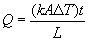# Conduction of Heat through a Material

• Jared944
In summary, the problem involves calculating the heat per second per square meter that is conducted through a combination of ice and aluminum with specified temperatures and thermal conductivities. Using the equation for heat conduction through a material, the interface temperature can be found and used to solve for the heat flux. The resulting answer should be in J/(s*m^2).

## Homework Statement

.005 m of Ice is in contact with .0015 m of Aluminum. The temp of the Ice is -10.0 degrees C, and the temp of the Aluminum is -25.0 degrees C.

I need to calculate the heat per second per square meter that is conducted through the Ice - Aluminum combination

## Homework Equations

The equation for the conduction of heat through a Material is (see attachment) Q= ((kA Change in T)t)/L

I know that the answer needs to be in J/(s*m^2), but I am unsure how to get to that point.

Thanks for any help!

Jared

#### Attachments

•formula.gif
245 bytes · Views: 553
I am assuming that the temperature is -10 C at the side of the ice away from the aluminum and the temperature is -25 C at the side of the aluminum away from the ice. The thermal conductivity of the ice is 2.34 W/m-K and the thermal conductivity of aluminum is 237 W/m-K in this temperature range. If T is the interface temperature between the ice and the aluminum, then $$q=2.34\frac{(-10-T)}{0.005}=237\frac{[T-(-25)]}{0.0015}$$where q is the heat flux.

If we solve for the interface temperature T, we obtain -24.956 C. This is very close to the aluminum temperature on the far boundary. Substituting into the equation gives a value of 7000 W/m^2 for the heat flux q.